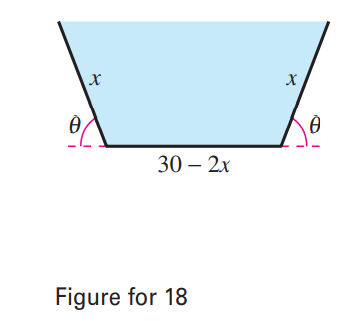×
Get Full Access to Calculus: Early Transcendental Functions - 6 Edition - Chapter 13.9 - Problem 18
Get Full Access to Calculus: Early Transcendental Functions - 6 Edition - Chapter 13.9 - Problem 18

×

# ?A trough with trapezoidal cross-sections is formed by turning up the edges of a 30-inch-wide sheet of aluminum (see figure). Find the cross-section ofISBN: 9781285774770 141

## Solution for problem 18 Chapter 13.9

Calculus: Early Transcendental Functions | 6th Edition

• Textbook Solutions
• 2901 Step-by-step solutions solved by professors and subject experts
• Get 24/7 help from StudySoup virtual teaching assistantsCalculus: Early Transcendental Functions | 6th Edition

4 5 1 326 Reviews
21
4
Problem 18

A trough with trapezoidal cross-sections is formed by turning up the edges of a 30-inch-wide sheet of aluminum (see figure). Find the cross-section of the maximum area.Step-by-Step Solution:

Step 1 of 5) Caution Theorem 7 does not say that gqn =1 an converges if an S 0. It is possible for a series to diverge when an S 0.

Step 2 of 2

##### ISBN: 9781285774770

This textbook survival guide was created for the textbook: Calculus: Early Transcendental Functions, edition: 6. Since the solution to 18 from 13.9 chapter was answered, more than 225 students have viewed the full step-by-step answer. The answer to “?A trough with trapezoidal cross-sections is formed by turning up the edges of a 30-inch-wide sheet of aluminum (see figure). Find the cross-section of the maximum area.” is broken down into a number of easy to follow steps, and 27 words. This full solution covers the following key subjects: . This expansive textbook survival guide covers 134 chapters, and 10738 solutions. The full step-by-step solution to problem: 18 from chapter: 13.9 was answered by , our top Calculus solution expert on 11/14/17, 10:53PM. Calculus: Early Transcendental Functions was written by and is associated to the ISBN: 9781285774770.

## Discover and learn what students are asking

Chemistry: The Central Science : Atoms, Molecules, and Ions
?Are these two compounds isomers? Explain. [Section 2.9]

Statistics: Informed Decisions Using Data : Comparing Three or More Means (One-Way Analysis of Variance)
?The variability within each treatment group, which is a weighted average of the sample variances from each treatment where the weights are based on th

Statistics: Informed Decisions Using Data : Inference about the Difference between Two Medians: Dependent Samples
?In Problems 3–10, use the Wilcoxon matched-pairs signedranks test to test the given hypotheses at the a = 0.05 level of significance. The dependent sa

Statistics: Informed Decisions Using Data : Inference about the Difference between Two Medians: Independent Samples
?In Problems 1–8, use the Mann–Whitney test to test the given hypotheses at the = 0.05 level of significance. The independent samples were obtained ra

Chemistry: The Central Science : The Chemistry of Life: Organic and Biological Chemistry
?Identify the carbon atom(s) in the structure shown that has (have) each of the following hybridizations: (a) s p^{3}, (b) sp,

Unlock Textbook Solution